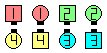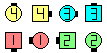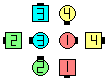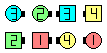Definitions of Square Dance Calls and Concepts

Index -->  Plus  |  A1  |  A2  |  C1  |  C2  |  C3A  |  C3B  |  C4  |  NOL  |
Definitions (Text Only) -->  Plus  |  A1  |  A2  |  C1  |  C2  |  C3A  |  C3B  |  C4  |  NOL  |
 Find call:

\$B8~\$+\$\$9g\$C\$?(B Line \$B\$^\$?\$OB>\$NE,@Z\$J(B formation \$B\$+\$i!%(B

Centers \$B\$O(B Touch 1/4 \$B\$r\$7\$F(B, Cross Concentric Vertical Tag The Line \$B\$r\$7(B, Lead \$B\$N?M\$O(B Peel Left, Trailer \$B\$N?M\$O(B Peel Right \$B\$r\$7\$F%+%C%W%k\$G=*\$o\$j\$^\$9(B (Sets In Motion \$B\$N\$h\$&\$K(B)\$B!%(B \$B\$=\$N4V\$K(B Outsides \$B\$O(B Circulate once and a half, Hinge, Cross Concentric Vertical 1/2 Tag \$B\$r\$7\$F(B, Face In \$B\$r\$7\$^\$9!%(B

\$B8~\$+\$\$9g\$C\$?(B Line \$B\$+\$i\$O(B Double Pass Thru \$B\$G=*\$o\$j\$^\$9!%(BPlan Ahead\$B\$NA0(BCenters Touch 1/4 as Ends Circulate\$B\$N8e(B Centers Vertical Tag The Line as Outsides 1/2 Circulate & Hinge\$B\$N8e(B Centers Step Forward & First Left, Next Right as Outsides Cross Concentric Vertical 1/2 Tag & Face In\$B\$N8e(B (\$B=*\$o\$j(B)

\$BCm
• Plan Ahead \$B\$O(B, \$B8~\$+\$\$9g\$C\$?(B Line \$B0J30\$N(B formation (\$BNc\$(\$P(B, ends \$B\$,30\$r8~\$\$\$?(B Inverted Lines) \$B\$+\$i9T\$o\$l\$^\$9(B: Ends \$B\$O(B Hinge \$B\$r\$9\$kA0\$N(B Circulate 1 & 1/2 \$B\$KCm0U\$9\$kI,MW\$,\$"\$j\$^\$9!%(B Ends \$B\$K\$O(B, \$B;O\$a\$K2q\$&?M\$H(B Hinge \$B\$r\$9\$k798~\$,\$"\$j\$^\$9!%(B
• \$B6a\$4\$m;d\$?\$A\$O(B, centers \$B\$N;O\$a\$N%Q!<%H\$O(B Touch 1/4 \$B\$NBe\$o\$j\$K(B 1/2 Circulate \$B\$r\$7\$F(B Hinge \$B\$H?.\$8\$k%3!<%i!<\$,\$\$\$k\$3\$H\$rCN\$j\$^\$7\$?!%\$=\$&\$9\$k\$H(B, \$BB>\$K\$\$\$/\$D\$+\$N;O\$a\$N(B formation \$B\$,\$"\$k\$3\$H\$K\$J\$j\$^\$9!%(B
•Choreography for Plan AheadComments? Questions? Suggestions?